# FINC314 CH6 Flashcards

Set Details Share
created 6 years ago by gn0179
4,223 views
Page to share:
Embed this setcancel
COPY
code changes based on your size selection
Size:
X

1

Risk that can be eliminated through diversification is called ______ risk.

unique

firm-specific

diversifiable

all of these options

D

2

The _______ decision should take precedence over the _____ decision.

asset allocation; stock selection

bond selection; mutual fund selection

stock selection; asset allocation

stock selection; mutual fund selection

A

3

Many current and retired Enron Corp. employees had their 401k retirement accounts wiped out when Enron collapsed because ________.

they had to pay huge fines for obstruction of justice

their 401k accounts were held outside the company

their 401k accounts were not well diversified

none of these options

C

4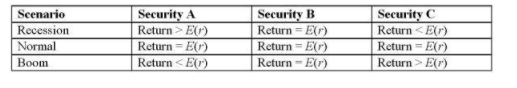Based on the outcomes in the following table, choose which of the statements below is (are) correct?

I. The covariance of security A and security B is zero.

II. The correlation coefficient between securities A and C is negative.

The correlation coefficient between securities B and C is positive.

I only

I and II only

II and III only

I, II, and III

B

5

Asset A has an expected return of 15% and a reward-to-variability ratio of .4. Asset B has an expected return of 20% and a reward-to-variability ratio of .3. A risk-averse investor would prefer a portfolio using the risk-free asset and ______.

asset A

asset B

no risky asset

The answer cannot be determined from the data given.

A

6

Adding additional risky assets to the investment opportunity set will generally move the efficient frontier _____ and to the ______.

up; right

up; left

down; right

down; left

B

7

An investor's degree of risk aversion will determine his or her ______.

optimal risky portfolio

risk-free rate

optimal mix of the risk-free asset and risky asset

capital allocation line

C

8

The ________ is equal to the square root of the systematic variance divided by the total variance.

covariance

correlation coefficient

standard deviation

reward-to-variability ratio

B

9

Which of the following statistics cannot be negative?

A. covariance
B. variance
C. E(r)
D. correlation coefficient

B

10

10. Asset A has an expected return of 20% and a standard deviation of 25%. The risk-free rate is 10%. What is the reward-to-variability ratio?

A. .40

B. .50

C. .75

D. .80

A

11

11. The correlation coefficient between two assets equals _________.

their covariance divided by the product of their variances

the product of their variances divided by their covariance

the sum of their expected returns divided by their covariance

their covariance divided by the product of their standard deviations

D

12

12. Diversification is most effective when security returns are _________.

high

negatively correlated

positively correlated

uncorrelated

B

13

13. The expected rate of return of a portfolio of risky securities is _________.

A. the sum of the securities' covariance
B. the sum of the securities' variance
C. the weighted sum of the securities' expected returns
D. the weighted sum of the securities' variance

C

14

14. Beta is a measure of security responsiveness to _________.

firm-specific risk

diversifiable risk

market risk

unique risk

C

15

15. The risk that can be diversified away is __________.

beta

firm-specific risk

market risk

systematic risk

B

16

16. Approximately how many securities does it take to diversify almost all of the unique risk from a portfolio?

2

6

8

20

D

17

17. Consider an investment opportunity set formed with two securities that are perfectly negatively correlated. The global minimum-variance portfolio has a standard deviation that is always _________.

equal to the sum of the securities' standard deviations

equal to -1

equal to 0

greater than 0

C

18

18. Market risk is also called __________ and _________.

systematic risk; diversifiable risk

systematic risk; nondiversifiable risk

unique risk; nondiversifiable risk

unique risk; diversifiable risk

B

19

19. Firm-specific risk is also called __________ and __________.

systematic risk; diversifiable risk

systematic risk; nondiversifiable risk

unique risk; nondiversifiable risk

unique risk; diversifiable risk

D

20

20. Which one of the following stock return statistics fluctuates the most over time?

A. covariance of returns
B. variance of returns
C. average return
D. correlation coefficient

C

21

21. Harry Markowitz is best known for his Nobel Prize-winning work on _____________.

techniques used to identify efficient portfolios of risky assets

techniques used to measure the systematic risk of securities

techniques used in valuing securities options

B

22

22. Suppose that a stock portfolio and a bond portfolio have a zero correlation. This means that ______.

the returns on the stock and bond portfolios tend to move inversely

the returns on the stock and bond portfolios tend to vary independently of each other

the returns on the stock and bond portfolios tend to move together

the covariance of the stock and bond portfolios will be positive

B

23

You put half of your money in a stock portfolio that has an expected return of 14% and a standard deviation of 24%. You put the rest of your money in a risky bond portfolio that has an expected return of 6% and a standard deviation of 12%. The stock and bond portfolios have a correlation of .55. The standard deviation of the resulting portfolio will be ________________.

more than 18% but less than 24%

equal to 18%

more than 12% but less than 18%

equal to 12%

C

24

24. On a standard expected return versus standard deviation graph, investors will prefer portfolios that lie to the _____________ the current investment opportunity set.

left and above

left and below

right and above

right and below

A

25

25. The term complete portfolio refers to a portfolio consisting of _________________.

the risk-free asset combined with at least one risky asset

the market portfolio combined with the minimum-variance portfolio

securities from domestic markets combined with securities from foreign markets

common stocks combined with bonds

A

26

26. Rational risk-averse investors will always prefer portfolios _____________.

located on the efficient frontier to those located on the capital market line

located on the capital market line to those located on the efficient frontier

at or near the minimum-variance point on the efficient frontier

that are risk-free to all other asset choices

B

27

27. The optimal risky portfolio can be identified by finding:

I. The minimum-variance point on the efficient frontier

II. The maximum-return point on the efficient frontier and the minimum-variance point on the efficient frontier

III. The tangency point of the capital market line and the efficient frontier

IV. The line with the steepest slope that connects the risk-free rate to the efficient frontier

I and II only

II and III only

III and IV only

I and IV only

C

28

28. The _________ reward-to-variability ratio is found on the ________ capital market line.

lowest; steepest

highest; flattest

highest; steepest

lowest; flattest

C

29

29. A portfolio is composed of two stocks, A and B. Stock A has a standard deviation of return of 24%, while stock B has a standard deviation of return of 18%. Stock A comprises 60% of the portfolio, while stock B comprises 40% of the portfolio. If the variance of return on the portfolio is

.0380, the correlation coefficient between the returns on A and B is _________.

A. .583

B. .225

C. .327

D. .128

A

30

30. The standard deviation of return on investment A is .10, while the standard deviation of return on investment B is .05. If the covariance of returns on A and B is .0030, the correlation coefficient between the returns on A and B is _________.

A. .12

B. .36

C. .60

D. .77

C

31

31. A portfolio is composed of two stocks, A and B. Stock A has a standard deviation of return of 35%, while stock B has a standard deviation of return of 15%. The correlation coefficient between the returns on A and B is .45. Stock A comprises 40% of the portfolio, while stock B comprises 60% of the portfolio. The standard deviation of the return on this portfolio is _________.

23%

19.76%

18.45%

17.67%

B

32

32. The standard deviation of return on investment A is .10, while the standard deviation of return on investment B is .04. If the correlation coefficient between the returns on A and B is -.50, the covariance of returns on A and B is _________.

-.0447

-.0020

.0020

.0447

B

33

33. Consider two perfectly negatively correlated risky securities, A and B. Security A has an expected rate of return of 16% and a standard deviation of return of 20%. B has an expected rate of return of 10% and a standard deviation of return of 30%. The weight of security B in the minimum-variance portfolio is _________.

10%

20%

40%

60%

C

34

34. An investor can design a risky portfolio based on two stocks, A and B. Stock A has an expected return of 18% and a standard deviation of return of 20%. Stock B has an expected return of 14% and a standard deviation of return of 5%. The correlation coefficient between the returns of A and B is .50. The risk-free rate of return is 10%. The proportion of the optimal risky portfolio that should be invested in stock A is _________.

0%

40%

60%

100%

A

35

An investor can design a risky portfolio based on two stocks, A and B. Stock A has an expected return of 18% and a standard deviation of return of 20%. Stock B has an expected return of 14% and a standard deviation of return of 5%. The correlation coefficient between the returns of A and B is .50. The risk-free rate of return is 10%. The expected return on the optimal risky portfolio is _________.

14%

15.6%

16.4%

18%

A

36

An investor can design a risky portfolio based on two stocks, A and B. Stock A has an expected return of 18% and a standard deviation of return of 20%. Stock B has an expected return of 14% and a standard deviation of return of 5%. The correlation coefficient between the returns of A and B is .50. The risk-free rate of return is 10%. The standard deviation of return on the optimal risky portfolio is _________.

0%

5%

7%

20%

B

37

An investor can design a risky portfolio based on two stocks, A and B. Stock A has an expected return of 21% and a standard deviation of return of 39%. Stock B has an expected return of 14% and a standard deviation of return of 20%. The correlation coefficient between the returns of A and B is .4. The risk-free rate of return is 5%. The proportion of the optimal risky portfolio that should be invested in stock B is approximately

_________.

29%

44%

56%

71%

D

38

An investor can design a risky portfolio based on two stocks, A and B. Stock A has an expected return of 21% and a standard deviation of return of 39%. Stock B has an expected return of 14% and a standard deviation of return of 20%. The correlation coefficient between the returns of A and B is .4. The risk-free rate of return is 5%. The expected return on the optimal risky portfolio is approximately _________. (Hint: Find weights first.)

14%

16%

18%

19%

B

39

39. An investor can design a risky portfolio based on two stocks, A and B. Stock A has an expected return of 21% and a standard deviation of return of 39%. Stock B has an expected return of 14% and a standard deviation of return of 20%. The correlation coefficient between the returns of A and B is .4. The risk-free rate of return is 5%. The standard deviation of returns on the optimal risky portfolio is _________.

25.5%

22.3%

21.4%

D. 20.7%

C

40

40. An investor can design a risky portfolio based on two stocks, A and B. The standard deviation of return on stock A is 24%, while the standard deviation on stock B is 14%. The correlation coefficient between the returns on A and B is .35. The expected return on stock A is 25%, while on stock B it is 11%. The proportion of the minimum-variance portfolio that would be invested in stock B is approximately _________.

45%

67%

85%

92%

C

41

41. An investor can design a risky portfolio based on two stocks, A and B. The standard deviation of return on stock A is 20%, while the standard deviation on stock B is 15%. The correlation coefficient between the returns on A and B is 0%. The rate of return for stocks A and B is 20 and 10 respectively. The expected return on the minimum-variance portfolio is approximately _________.

10%

13.6%

15%

19.41%

B

42

42. An investor can design a risky portfolio based on two stocks, A and B. The standard deviation of return on stock A is 20%, while the standard deviation on stock B is 15%. The correlation coefficient between the returns on A and B is 0%. The standard deviation of return on the minimum-variance portfolio is _________.

0%

6%

12%

17%

C

43

43. A measure of the riskiness of an asset held in isolation is ____________.

beta

standard deviation

covariance

alpha

B

44

44. Semitool Corp. has an expected excess return of 6% for next year. However, for every unexpected 1% change in the market, Semitool's return responds by a factor of 1.2. Suppose it turns out that the economy and the stock market do better than expected by 1.5% and Semitool's products experience more rapid growth than anticipated, pushing up the stock price by another 1%. Based on this information, what was Semitool's actual excess return?

7%

8.5%

8.8%

9.25%

C

45

45. The part of a stock's return that is systematic is a function of which of the following variables?

I. Volatility in excess returns of the stock market

II. The sensitivity of the stock's returns to changes in the stock market

The variance in the stock's returns that is unrelated to the overall stock market

I only

I and II only

II and III only

I, II, and III

B

46

46. Stock A has a beta of 1.2, and stock B has a beta of 1. The returns of stock A are ______ sensitive to changes in the market than are the returns of stock B.

20% more

slightly more

20% less

slightly less

A

47

47. Which risk can be partially or fully diversified away as additional securities are added to a portfolio?

I. Total risk

II. Systematic risk

Firm-specific risk

I only

I and II only

I, II, and III

I and III

D

48

48. According to Tobin's separation property, portfolio choice can be separated into two independent tasks consisting of __________ and

__________.

A.identifying all investor imposed constraints; identifying the set of securities that conform to the investor's constraints and offer the best risk-return trade-offs
B.identifying the investor's degree of risk aversion; choosing securities from industry groups that are consistent with the investor's risk profile

C.identifying the optimal risky portfolio; constructing a complete portfolio from T-bills and the optimal risky portfolio based on the investor's degree of risk aversion
D.choosing which risky assets an investor prefers according to the investor's risk-aversion level; minimizing the CAL by lending at the risk-free rate

C

49

You are constructing a scatter plot of excess returns for stock A versus the market index. If the correlation coefficient between stock A and the index is -1, you will find that the points of the scatter diagram ___________ and the line of best fit has a ______________.

all fall on the line of best fit; positive slope

all fall on the line of best fit; negative slope

are widely scattered around the line; positive slope

are widely scattered around the line; negative slope

B

50

50. The term excess return refers to ______________.

returns earned illegally by means of insider trading

the difference between the rate of return earned and the risk-free rate

the difference between the rate of return earned on a particular security and the rate of return earned on other securities of equivalent risk

the portion of the return on a security that represents tax liability and therefore cannot be reinvested

B

51

51. You are recalculating the risk of ACE stock in relation to the market index, and you find that the ratio of the systematic variance to the total variance has risen. You must also find that the ____________.

covariance between ACE and the market has fallen

correlation coefficient between ACE and the market has fallen

correlation coefficient between ACE and the market has risen

unsystematic risk of ACE has risen

C

52

52. A stock has a correlation with the market of .45. The standard deviation of the market is 21%, and the standard deviation of the stock is 35%. What is the stock's beta?

A. 1

B. .75

C. .60

D. .55

B

53

53. The values of beta coefficients of securities are __________.

always positive

always negative

always between positive 1 and negative 1

usually positive but are not restricted in any particular way

D

54

54. A security's beta coefficient will be negative if ____________.

its returns are negatively correlated with market-index returns

its returns are positively correlated with market-index returns

its stock price has historically been very stable

market demand for the firm's shares is very low

A

55

55. The market value weighted-average beta of firms included in the market index will always be _____________.

0

between 0 and 1

1

none of these options (There is no particular rule concerning the average beta of firms included in the market index.)

C

56

56. Diversification can reduce or eliminate __________ risk.

all

systematic

nonsystematic

D. only an insignificant

C

57

57. To construct a riskless portfolio using two risky stocks, one would need to find two stocks with a correlation coefficient of ________.

A. 1

B. .5

0

-1

D

58

58. Some diversification benefits can be achieved by combining securities in a portfolio as long as the correlation between the securities is

_____________.

1

less than 1

between 0 and 1

less than or equal to 0

B

59

59. If an investor does not diversify his portfolio and instead puts all of his money in one stock, the appropriate measure of security risk for that investor is the ________.

stock's standard deviation

variance of the market

stock's beta

covariance with the market index

A

60

60. Which of the following provides the best example of a systematic-risk event?

A strike by union workers hurts a firm's quarterly earnings.

Mad Cow disease in Montana hurts local ranchers and buyers of beef.

The Federal Reserve increases interest rates 50 basis points.

A senior executive at a firm embezzles \$10 million and escapes to South America.

C

61

61. Which of the following statements is (are) true regarding time diversification?

I. The standard deviation of the average annual rate of return over several years will be smaller than the 1-year standard deviation.

II. For a longer time horizon, uncertainty compounds over a greater number of years.

Time diversification does not reduce risk.

I only

II only

II and III only

I, II, and III

C

62

62. You find that the annual Sharpe ratio for stock A returns is equal to 1.8. For a 3-year holding period, the Sharpe ratio would equal _______.

1.8

2.48

3.12

5.49

C

63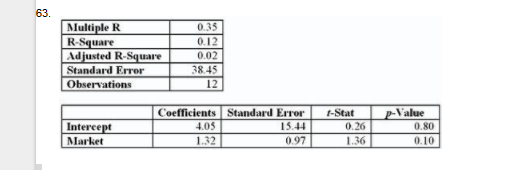The beta of this stock is ____.

A. .12

B. .35

1.32

4.05

C

64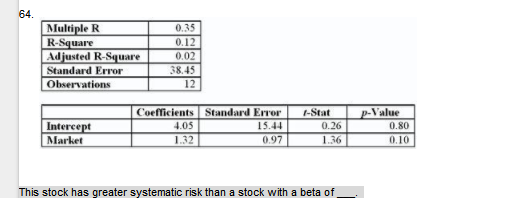This stock has greater systematic risk than a stock with a beta of ___.

A. .50

1.5

2

3

A

65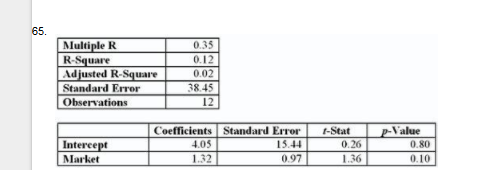The characteristic line for this stock is Rstock = ___ + ___ Rmarket.

A. .35; .12

4.05; 1.32

15.44; .97

D. 26; 1.36

B

66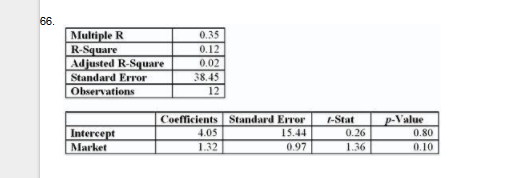_______________ % of the variance is explained by this regression.

12

35

4.05

80

A

67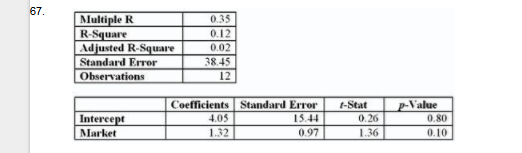The stock is ______ riskier than the typical stock.

32%

15.44%

12%

38%

A

68

68. Decreasing the number of stocks in a portfolio from 50 to 10 would likely ________________.

increase the systematic risk of the portfolio

increase the unsystematic risk of the portfolio

increase the return of the portfolio

decrease the variation in returns the investor faces in any one year

B

69

69. If you want to know the portfolio standard deviation for a three-stock portfolio, you will have to ______.

calculate two covariances and one trivariance

calculate only two covariances

calculate three covariances

average the variances of the individual stocks

C

70

70. Which of the following correlation coefficients will produce the least diversification benefit?

-.6

-.3

0

.8

D

71

71. Which of the following correlation coefficients will produce the most diversification benefits?

-.6

-.9

0

.4

B

72

72. What is the most likely correlation coefficient between a stock-index mutual fund and the S&P 500?

-1

0

1

.5

C

73

73. Investing in two assets with a correlation coefficient of -.5 will reduce what kind of risk?

A. market risk
B. nondiversifiable risk
C. systematic risk
D. unique risk

D

74

74. Investing in two assets with a correlation coefficient of 1 will reduce which kind of risk?

A. market risk
B. unique risk
C. unsystematic risk
D. none of these options (With a correlation of 1, no risk will be reduced.)

D

75

75. A portfolio of stocks fluctuates when the Treasury yields change. Since this risk cannot be eliminated through diversification, it is called

__________.

firm-specific risk

systematic risk

unique risk

none of the options

B

76

76. As you lengthen the time horizon of your investment period and decide to invest for multiple years, you will find that:

I. The average risk per year may be smaller over longer investment horizons.

II. The overall risk of your investment will compound over time.

Your overall risk on the investment will fall.

I only

I and II only

III only

I, II, and III

B

77

. You are considering adding a new security to your portfolio. To decide whether you should add the security, you need to know the security's:

I. Expected return

II. Standard deviation

I only

I and II only

I and III only

I, II, and III

D

78

Which of the following is a correct expression concerning the formula for the standard deviation of returns of a two-asset portfolio where the correlation coefficient is positive?

A. σ2rp < (W12σ12 + W22σ22)
B. σ2rp = (W12σ12 + W22σ22)
C. σ2rp = (W12σ12 - W22σ22)
D. σ2rp > (W12σ12 + W22σ22)

D

79

79. What is the standard deviation of a portfolio of two stocks given the following data: Stock A has a standard deviation of 18%. Stock B has a standard deviation of 14%. The portfolio contains 40% of stock A, and the correlation coefficient between the two stocks is -.23.

9.7%

12.2%

14%

15.6%

A

80

80. What is the standard deviation of a portfolio of two stocks given the following data: Stock A has a standard deviation of 30%. Stock B has a standard deviation of 18%. The portfolio contains 60% of stock A, and the correlation coefficient between the two stocks is -1.

0%

10.8%

18%

24%

B

81

81. The expected return of a portfolio is 8.9%, and the risk-free rate is 3.5%. If the portfolio standard deviation is 12%, what is the reward-to-variability ratio of the portfolio?

A. 0

B. .45

C. .74

D. 1.35

B

82

82. A project has a 60% chance of doubling your investment in 1 year and a 40% chance of losing half your money. What is the standard deviation of this investment?

25%

50%

62%

73%

D

83

83. A project has a 50% chance of doubling your investment in 1 year and a 50% chance of losing half your money. What is the expected return on this investment project?

0%

25%

50%

75%

B

84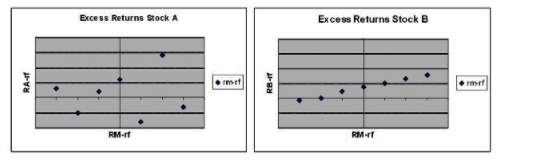84. The figures below show plots of monthly excess returns for two stocks plotted against excess returns for a market index.

Which stock is likely to further reduce risk for an investor currently holding her portfolio in a well-diversified portfolio of common stock?

Stock A

Stock B

There is no difference between A or B.

The answer cannot be determined from the information given.

A

85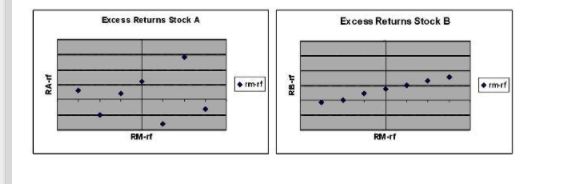85. The figures below show plots of monthly excess returns for two stocks plotted against excess returns for a market index.

Which stock is riskier to a nondiversified investor who puts all his money in only one of these stocks?

Stock A is riskier.

Stock B is riskier.

Both stocks are equally risky.

The answer cannot be determined from the information given.

A

86

86. In the article “Danger: High Levels of Company Stock,” what is the maximum amount of your employer’s stock that the author recommends you hold in your retirement account?

A. 5%
B. 10%
C. 50%
D. 90%

B

87

87. The efficient frontier represents a set of portfolios that

A. maximize expected return for a given level of risk.
B. minimize expected return for a given level of risk.
C. maximize risk for a given level of return.
D. None of the options.

A

88

88. The portfolio with the lowest standard deviation for any risk premium is called the_______.

A. CAL portfolio
B. efficient frontier portfolio
C. global minimum variance portfolio
D. optimal risky portfolio

C

89

89. Lear Corp. has an expected excessreturn of 8% next year. Assume Lear’s beta is 1.43. If the economy booms and the stock market beats expectations by 5%, what was Lear’s actual excess return?

A. 7.15%
B. 13%
C. 15.15%
D. 18.59 %

C

90

90. The plot of a security’s excess return relative to the market’s excess return is called the _______.

A. efficient frontier
B.security characteristic line
C. capital allocation line
D. capital market line

B